### Home > CALC > Chapter 8 > Lesson 8.1.4 > Problem8-43

8-43.
1. Differentiate. Homework Help ✎

1.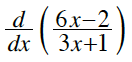2.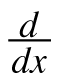(sec(5 ln x))

3.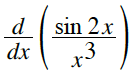4.(k293k−1)

5.(sin2x cos x)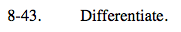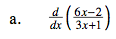Quotient Rule.

$\frac{d}{dx}\text{sec}x=\text{sec}x\text{tan}x$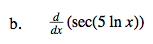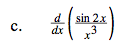$=\frac{2x^{3}\text{cos}(2x)-3x^{2}\text{sin}(2x)}{x^{6}}$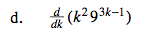We know that k is a variable (not a constant) because it appears in the

$\frac{d}{dk}.$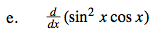Product Rule.